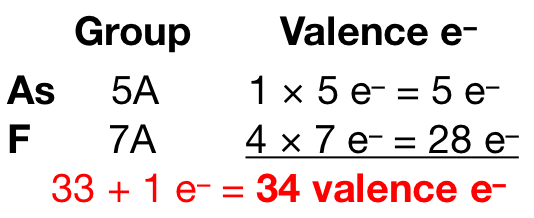# Problem: These species do not obey the octet rule. Draw a Lewis structure for each, and state the type of octet-rule exception: (b) AsF4 −

###### FREE Expert Solution

Recall: An atom in a molecule that does not obey the octet rule either

1. has an odd number of electrons
2. has less than an octet of electrons
3. has more than an octet of electrons

Arsenic (EN = 2.18) is less electronegative than Fluorine (EN = 3.98) so As is the central atom. We first need to calculate the total number of valence electrons in the molecule:94% (186 ratings)###### Problem Details

These species do not obey the octet rule. Draw a Lewis structure for each, and state the type of octet-rule exception:

(b) AsF4